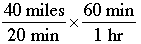SEARCH HOMEMath Central Quandaries & QueriesQuestion from ron, a parent: how fast am i going if i drive 40 miles in 20 minutes...what is the formula for figuring this out.Hi Ron,

To calculate a speed one always divides distance traveled by the time it took to cover that distance. Depending on the units you would like to report the speed in (miles per hour, metres per second etc.), this calculation may also require that you convert from one unit of measurement to another.

If you travel 40 miles in 20 minutes, your speed in miles per minute can be calculated as: 2 miles per minute.

If you want miles per hour, there are a number of ways to calculate this, but perhaps the easiest is to consider: if I go 40 miles in 20 minutes, then I would go 80 miles in 40 minutes, and thus 120 in 60 minutes, which is one hour. In other words, there are three 20 minute intervals in one hour, each of which will result in a distance of 40 miles.

If you insist on a formula (which is ok, because the formula would work for any distance and time combination), there are two options:

1. Calculate the speed in miles per minute and then multiply by 60 minutes per hour. The unit "minutes" is eliminated by this calculation and "hours" is inserted in its place.

2. Set up a speed ratio in the units given (miles and minutes) and multiply by the "minutes to hours" ratio:= speed in miles per hour

Hope this helps,
LeeanneMath Central is supported by the University of Regina and The Pacific Institute for the Mathematical Sciences.